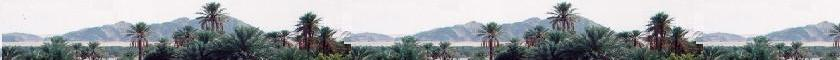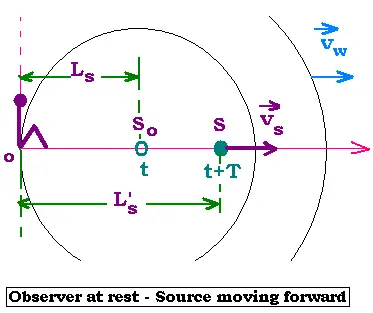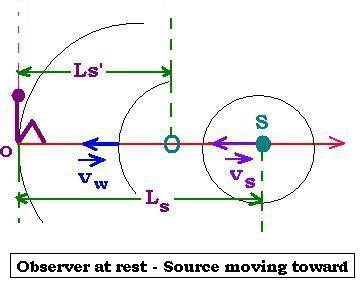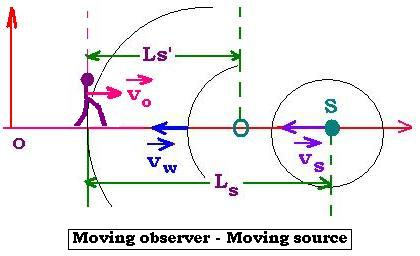##### Contents

A sigle wave

Superposition of waves

# Doppler effect

### 1. Moving source forward an observer at restThe source S is moving at right at the speed Vs in the frame of the observer at rest. At the precise time T, which is the period of the wave; this wave that was emitted from So reaches the observer. The source at this time is not located at the position So, that is at the distance Ls, but It was moved and now It is located at the distance L's. For the observer, It appears that the wave was originated from the position S taking T' as time to arrive at its position. If Vwis the speed of the wave, we can write:
L's = Vw. T'
We have:
Ls = Vw. T
And:
L's = Ls + Vs. T
Thus:
Vw. T '= Vw. T +Vs. T
Then:
T' = T . (Vw+ Vs)/Vw
With ƒ = 1/T, we have:
ƒ' = ƒ[Vw/(Vw+ Vs)]
ƒobs = ƒ . Vw/(Vw+ Vs)
Where ƒ' is the apparent frequency for the Observer, and ƒ is the real frequency of the wave.
If the source is stationary (Vs = 0), then ƒ' = ƒ' and T' = T, that is the observer gets waves at every real period.

### 2. Moving source toward an observer at restNext, the observer is always at rest and the source S is moving at a speed Vs towards this observer. The wave propagates at the speed Vwin all directions and towards the observer of course. When this observer receives the wave, at this precise time, the source is not located at the distance Ls but at L's. For the observer, the period of the wave is T's. And at this precise time, the wave has travelled Ls = Vw. T , where T is the real period . T's is the apparent period.
We can write:
L's = Ls - Vs . T
Because Ls = Vw. T
and L's = Vw. T's
Thus :
Vw. T's = Vw. T - Vs . T = (Vw- Vs) . T Or:
1/T's = Vw/(Vw- Vs) (1/T)
ƒ' = Vw/(Vw- Vs) ƒ
ƒobs = ƒ . Vw/(Vw- Vs)
ƒobs is the apparent frequency.

### 3. Moving source and observerNow, let's consider that the observer is moving at a speed Vo and the sourse is moving at a speed Vs, oppraching the observer, that is in the opposite direction. The wave is always moving at the velocity Vw.

This case is like the last one except that the observer is moving. Then insted of L's = Vw. T' , we have:
L's = (Vw + Vo) . T'
Thus:
(Vw + Vo) . T' = (Vw- Vs) . T
Hence:

ƒ'=(Vw+Vo)/(Vw-Vs
ƒobs = ƒ . (Vw+Vo)/(Vw-Vs)

### 4. Moving source and observer : Relativistic case

In the relativistic case, the time is streched, that is the time T becomes γT, where
γ = 1/[1- (Vs/Vw)2]1/2.
Hence the frequency (1/T) is changed into: 1/γT. That is:
ƒ' (Relativistic) = ƒ'(Classic)/γ
For the latter case, we have:
ƒ'(Relativistic) = (Vw+Vo)/(Vw-Vs)ƒ/γ = (Vw+Vo)/(Vw-Vs) [1- (Vs/Vw)2]1/2 ƒ = ƒ . [(Vw+Vs)/(Vw-Vs)]1/2 . (Vw+Vo)/Vw.
ƒ' = ƒ . [(Vw+Vs)/(Vw-Vs)]1/2 . (Vw+Vo)/Vw
ƒobs = ƒ.[(Vw+Vs)/(Vw-Vs)]1/2 . (Vw+Vo)/Vw

In the case of the wave is light, Vw= c (speed of light in the vacuum = 3.10 8m/s) Then :

ƒ' = ƒ . ((c + Vs)/(c - Vs))1/2 . (c + Vo)/c
In the case of the observer is at rest, V0 = 0, then:
ƒ' = ƒ . [(c + Vs)/(c - Vs)]1/2.
ƒ' = ƒ . (1/c)(c + Vo)/[1 - (Vo/c)2)]1/2 ≈ ƒ(1 + Vo/c)
ƒobs = ƒ (1/c)(c + Vo)/[1 - (Vo/c)2)]1/2 ≈ ƒ(1 + Vo/c)